@yangfch3 2016-01-20T04:54:44.000000Z 字数 4733 阅读 4207

# 线性代数

化学

## 行列式的性质

${\\ D= \left| \begin{matrix} a_{11}&...&a_{1i}+a_{1i}^{'}&a_{1n}\\\\ a_{21}&...&a_{2i}+a_{2i}^{'}&a_{2n}\\\\ ...&...&...&...\\\\ a_{n1}&...&a_{ni}+a_{ni}^{'}&a_{nn}\\\\ \end{matrix} \right| \\}$

${\\ D= {\left| \begin{matrix} a_{11}&...&a_{1i}&a_{1n}\\\\ a_{21}&...&a_{2i}&a_{2n}\\\\ ...&...&...&...\\\\ a_{n1}&...&a_{ni}&a_{nn}\\\\ \end{matrix} \right|} + {\left| \begin{matrix} a_{11}&...&a_{1i}^{'}&a_{1n}\\\\ a_{21}&...&a_{2i}^{'}&a_{2n}\\\\ ...&...&...&...\\\\ a_{n1}&...&a_{ni}^{'}&a_{nn}\\\\ \end{matrix} \right|} \\}$

## 二阶、三阶行列式的运算

$\left| \begin{matrix} a_{11}&a_{12}\\\\ a_{21}&a_{22}\\\\ \end{matrix} \right| =a_{11}a_{22}-a_{12}a_{21}$

$\left| \begin{matrix} a_{11}&a_{12}&a_{13}\\\\ a_{21}&a_{22}&a_{23}\\\\ a_{31}&a_{32}&a_{33}\\\\ \end{matrix} \right| =a_{11}a_{22}a_{33}+a_{12}a_{23}a_{31}+a_{13}a_{21}a_{32}-a_{13}a_{22}a_{31}-a_{12}a_{21}a_{33}-a_{11}a_{23}a_{32}$

## 高阶行列式运算

1. 定义法求解
行列式有多少项：全排列
每一项前面的正负号：逆序数（某个数的前面比它大的数的个数）
偶排列(+) 基排列(-)
对换相邻两个元素，排列改变奇偶性.

2. 变换法求解：三角型行列式
$上三角行列式(下三角同理): {\\ \left| \begin{matrix} a_{11}&a_{12}&a_{13}&a_{14}\\\\ 0&a_{22}&a_{23}&a_{24}\\\\ 0&0&a_{33}&a_{34}\\\\ 0&0&0&a_{44}\\\\ \end{matrix} \right| =a_{11}a_{22}a_{33}a_{44} \\} 对角线行列式： {\\ \left| \begin{matrix} λ_1&&&\\\\ &λ_2&&\\\\ &&...&\\\\ &&&λ_n\\\\ \end{matrix} \right| =λ_1λ_2...λ_n } {\\ \left| \begin{matrix} &&&λ_1\\\\ &&λ_2&\\\\ &...&&\\\\ λ_n&&&\\\\ \end{matrix} \right| =(-1)^{\frac {n(n-1)}2}λ_1λ_2...λ_n }$

3. 展开法求解：代数余子式
余子式　在n阶行列式中，把元素$a_{ij}$所在的第i行和第j列划去后，留下来的n-1阶行列式叫做元素$a_{ij}$的余子式，记作$M_{ij}$.
代数余子式　$A_{ij}=(-1)^{i+j}M_{ij}$

$D=a_{i1}A_{i1}+a_{i2}A_{i2}+...+a_{in}A_{in}$

$D=a_{ij}A_{ij}$

## 线性方程组求解

1. 线性方程组解的判据
${\\齐次线性方程组Ax=0\\ R(A)=n\Leftrightarrow 只有零解\\ R(A)＜n\Leftrightarrow 有非零解\\} {\\非齐次线性方程组Ax=b\\ R(A)=R(B)\Leftrightarrow 有解\\ R(A)=R(B)=nÛ\Leftrightarrow 有唯一解\\ R(A)=R(B)＜nÛ\Leftrightarrow 有无穷多解}$
（其中，A为系数矩阵，B为增广矩阵，R为矩阵的秩，n为未知数的个数）

2. 消元法

3. 克莱姆法则

${ \left\{ \begin{array}{c} a_{11}x_1+a_{12}x_2+a_{13}x_3=b_1\\ \\ a_{21}x_1+a_{22}x_2+a_{23}x_3=b_2\\ \\ a_{31}x_1+a_{32}x_2+a_{33}x_3=b_3 \end{array} \right. } { D= \left| \begin{matrix} a_{11}&a_{12}&a_{13}\\\\ a_{21}&a_{22}&a_{23}\\\\ a_{31}&a_{32}&a_{33}\\\\ \end{matrix} \right| }$

${ D_1= \left| \begin{matrix} b_1&a_{12}&a_{13}\\\\ b_2&a_{22}&a_{23}\\\\ b_3&a_{32}&a_{33}\\\\ \end{matrix} \right| } { D_2= \left| \begin{matrix} a_{11}&b_1&a_{13}\\\\ a_{21}&b_2&a_{23}\\\\ a_{31}&b_3&a_{33}\\\\ \end{matrix} \right| } { D_3= \left| \begin{matrix} a_{11}&a_{12}&b_1\\\\ a_{21}&a_{22}&b_2\\\\ a_{31}&a_{32}&b_3\\\\ \end{matrix} \right| }$

$(D≠0，方程有唯一解) \\ {x_1=\frac {D_1}D} {x_1=\frac {D_2}D} {x_1=\frac {D_3}D}$

## 矩阵的运算

• 加减法
• 数乘
• 矩阵乘矩阵
$AB≠BA，(AB)^k≠A^kB^k$
• 转置矩阵
$(A+B)^T=A^T+B^T；(λA)^T=λA^T；(AB)^T=B^TA^T$
• 伴随矩阵
行列式$|A|$的各个元素$A_{ij}$的代数余子式所构成的矩阵的转置，称为矩阵$A$的伴随矩阵，记作$A^*$.
• 对称矩阵
$A=A^T$

## 逆矩阵

1. 逆矩阵的性质

• $若A可逆，则A^{-1}亦可逆，且(A^{-1})^{-1}=A$
• $若A可逆，数λ≠0，则λA可逆，且(λA)^{-1}=λ^{-1}A^{-1}$
• $若A，B为同阶方阵且均可逆，则AB亦可逆，且(AB)^{-1}=B^{-1}A^{-1}$
• $若A可逆，则A^T亦可逆，且(A^T)^{-1}=(A^{-1})^T$
• $若A可逆，则有|A^{-1}|=|A|^{-1}$
2. 利用代数余子式求逆阵
$A^{-1}=\frac 1{|A|}A^*\\ 故矩阵可逆的重要条件是|A|≠0.$

3. 待定系数法求逆阵
$已知矩阵A可逆，设B= \left( \begin{matrix} a&b\\\\ c&d\\\\ \end{matrix} \right)， 令AB=E，可求得A^{-1}.$
4. 消元法求逆阵
• 求方程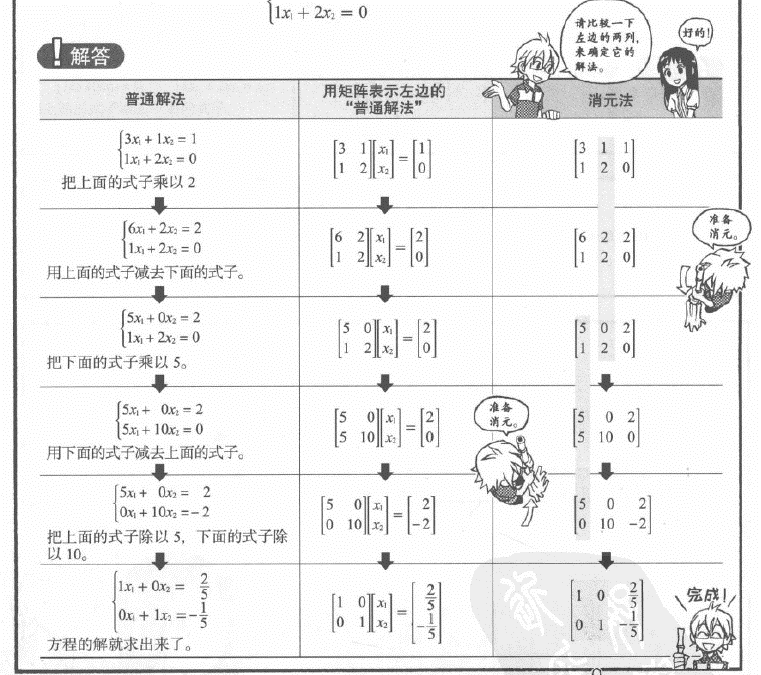• 求逆阵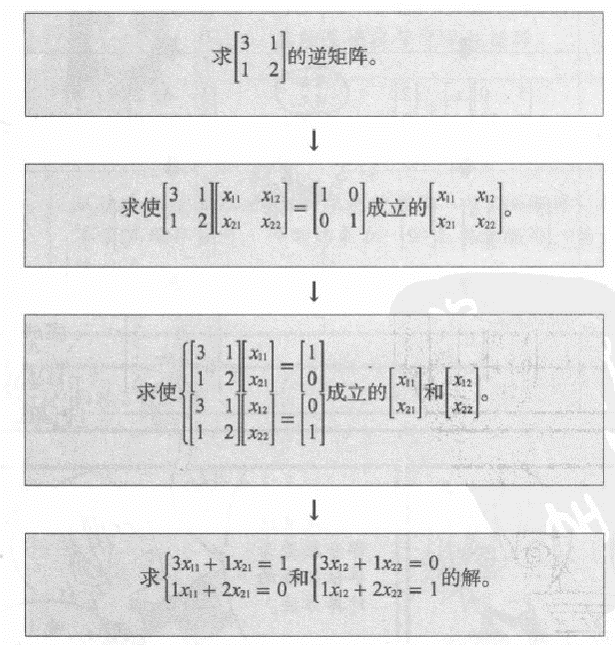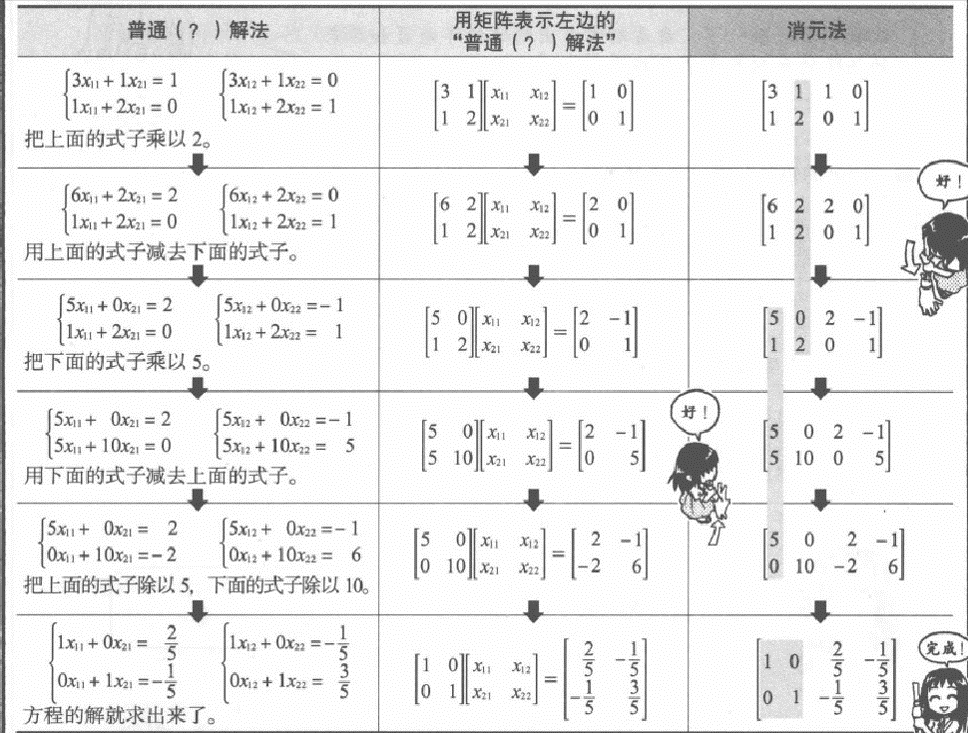5. 初等变换法

## 矩阵的初等变换

1. 定义
• 对调两行（列）；
• 以数$k≠0$乘某一行（列）的所有元素；
• 把某一行（列）所有元素的k倍加到另一行（列）对应的元素上去.
2. 初等矩阵
由单位矩阵$E$经过一次初等变换得到的方阵称为初等矩阵.

3. 初等变换
例如：
$\\ B= \left( \begin{matrix} 2&-1&-1&1&2\\\\ 1&1&-2&1&4\\\\ 4&-6&2&-2&4\\\\ 3&6&-9&7&9\\\\ \end{matrix} \right) {r_1\leftrightarrow r_2\over r_3÷2} \left( \begin{matrix} 1&1&-2&1&4\\\\ 2&-1&-2&2&2\\\\ 2&-3&5&-3&2\\\\ 3&6&-3&4&9\\\\ \end{matrix} \right)=B_1\\ B_1{{r_2-r_3，r_3-2r_1}\over r_4-3r_1} \left( \begin{matrix} 1&1&-2&1&4\\\\ 0&2&-2&2&0\\\\ 0&-5&5&-3&-6\\\\ 0&3&-3&4&-3\\\\ \end{matrix} \right) {{r_2÷2，r_3+5r_2}\over r_4-3r_2} \left( \begin{matrix} 1&1&-2&1&4\\\\ 0&1&-1&1&0\\\\ 0&0&0&2&-6\\\\ 0&0&0&1&-3\\\\ \end{matrix} \right)=B_3\\ B_3{r_3\leftrightarrow r_4\over r_4-2r_3} \left( \begin{matrix} 1&1&-2&1&4\\\\ 0&1&-1&1&0\\\\ 0&0&0&1&-3\\\\ 0&0&0&0&0\\\\ \end{matrix} \right)=B_4 {r_1-r_2\over r_2-2r_3} \left( \begin{matrix} 1&0&-1&0&4\\\\ 0&1&-1&0&3\\\\ 0&0&0&1&-3\\\\ 0&0&0&0&0\\\\ \end{matrix} \right)=B_5\\$
其中$B_4$$B_5$都称为行阶梯形矩阵

• 可划出一条阶梯线，线的下方全为零；
• 每个台阶只有一行，台阶数是非零行数；
• 对于任何矩阵$A_{m×n}$总可经过有限次初等行变换得到行阶梯形和行最简形.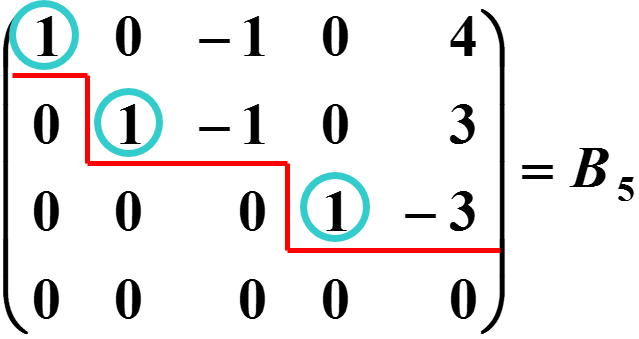$B_5$还称为行最简形矩阵，非零行的第一个非零元为1.
$B_5$再经过列变换得到$F$$F$称为$B$标准形.## 矩阵的秩

1. 秩的用处：

• 向量的线性相关性-哪些向量相互表示；
• 方程组解的形式-多少个通解；
• 环境中独立的自由变量.
2. 秩的性质：
$转置后秩不变\\ r(A)≤min(m,n),A是m*n型矩阵\\ r(kA)=r(A),k不等于0\\ r(A)=0 \Leftrightarrow A=0\\ r(A+B)≤r(A)+r(B)\\ r(AB)≤min(r(A),r(B))\\ r(A)+r(B)-n≤r(AB)$

## 矩阵的分块

1. 定义
将矩阵$A$用若干条纵线和横线分成许多个小矩阵，每一个小矩阵称为$A$子块，以子块为元素的形式上的矩阵称为分块矩阵.

2. 分块矩阵的运算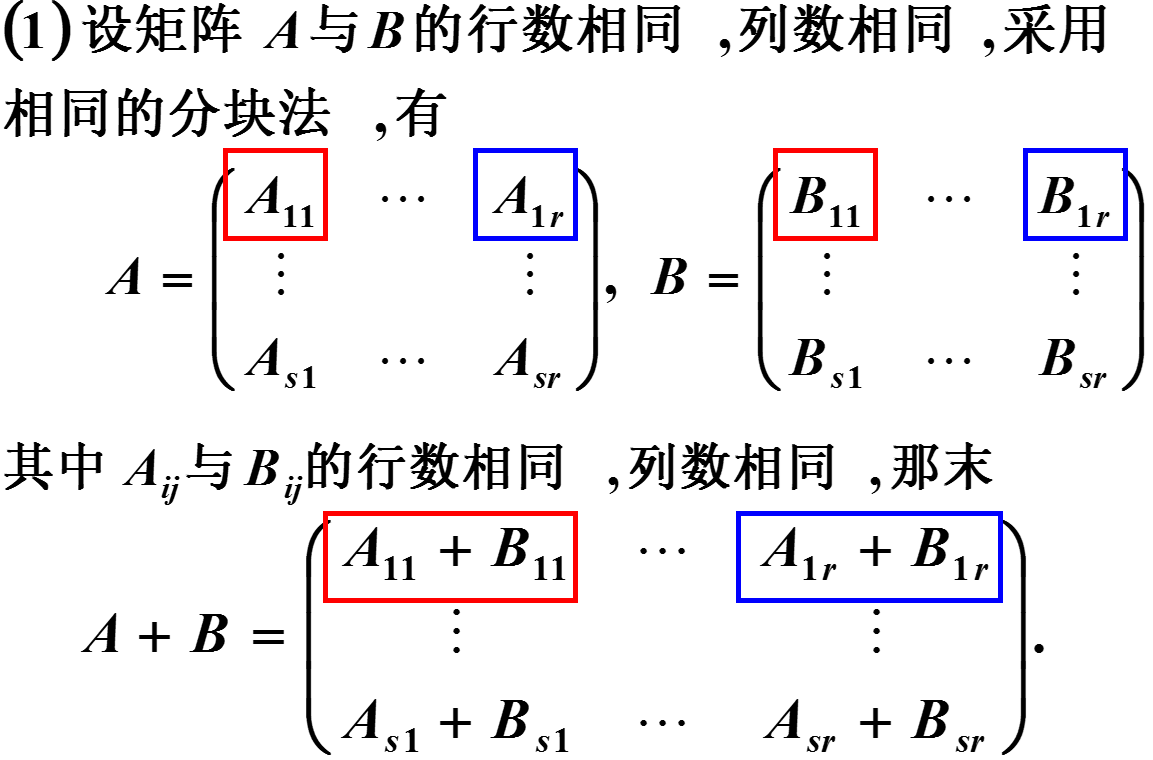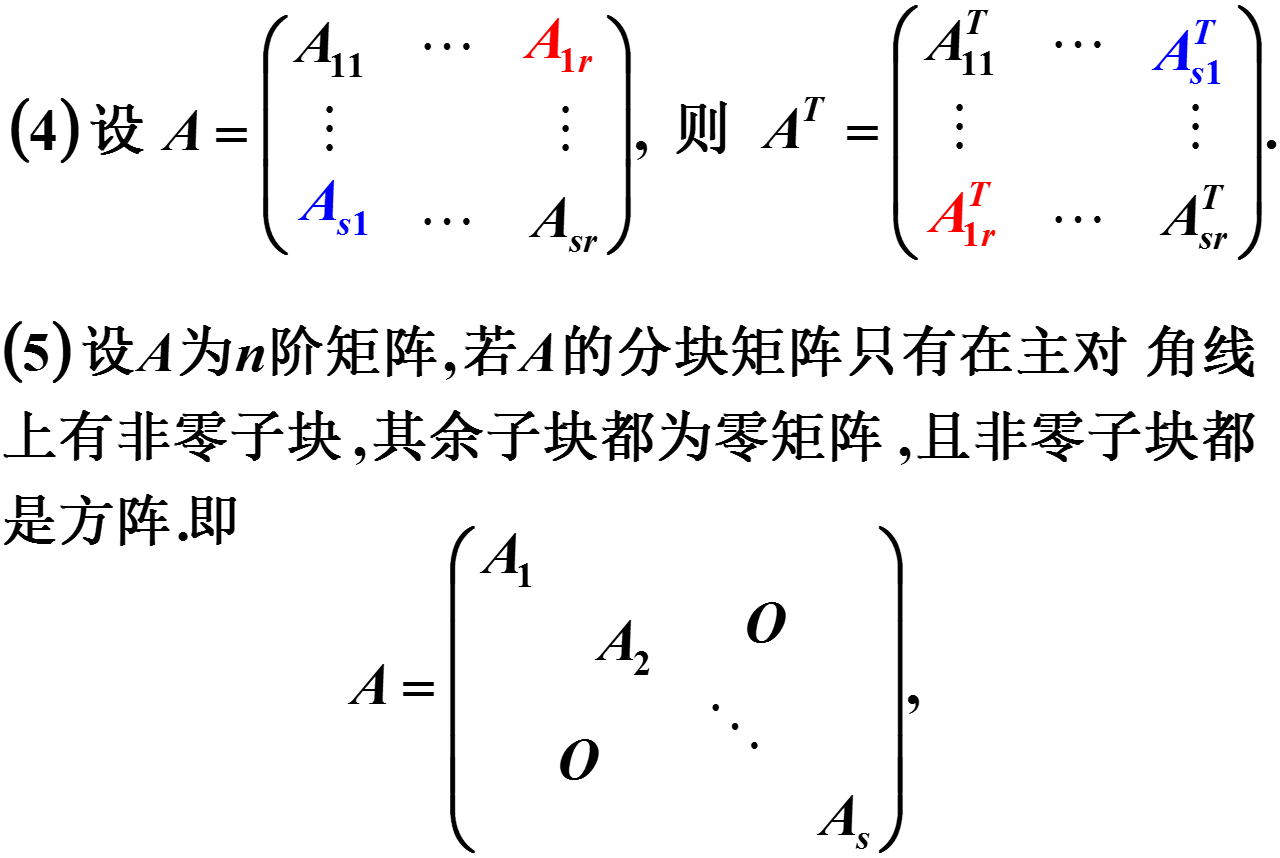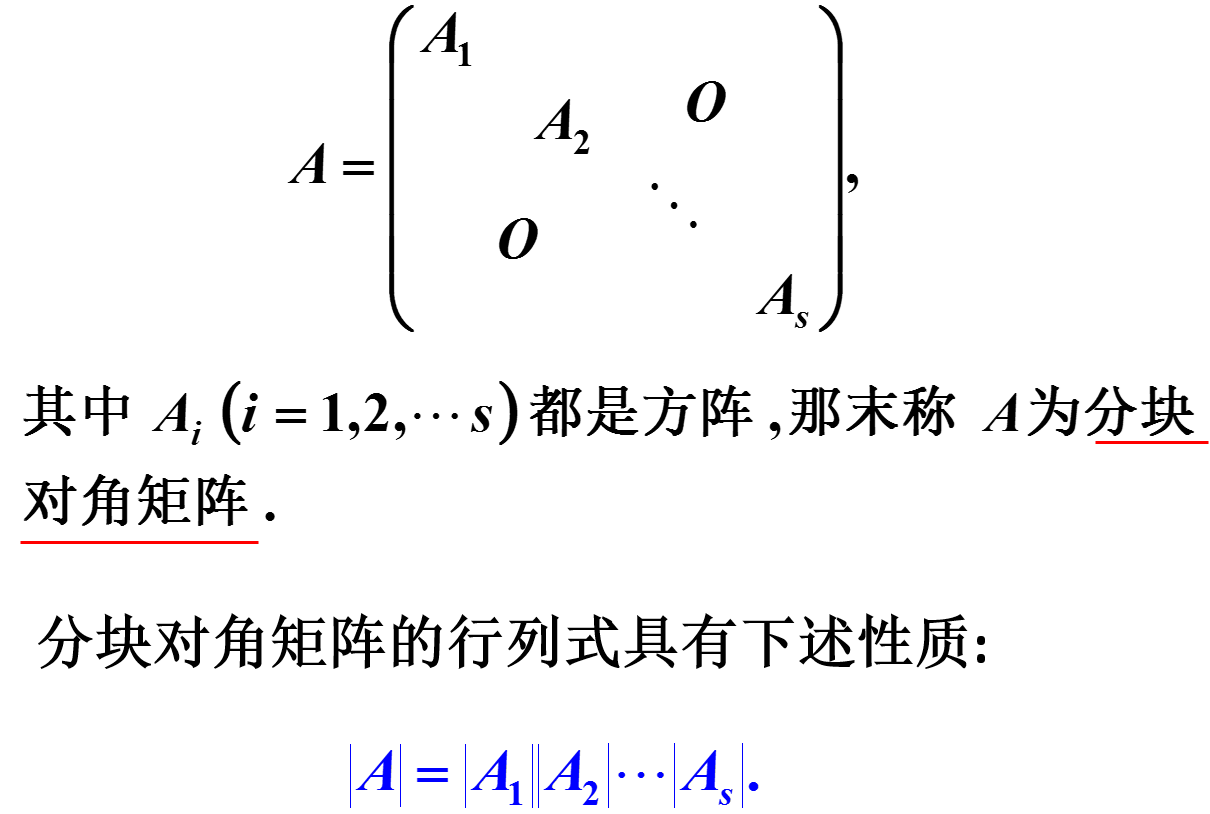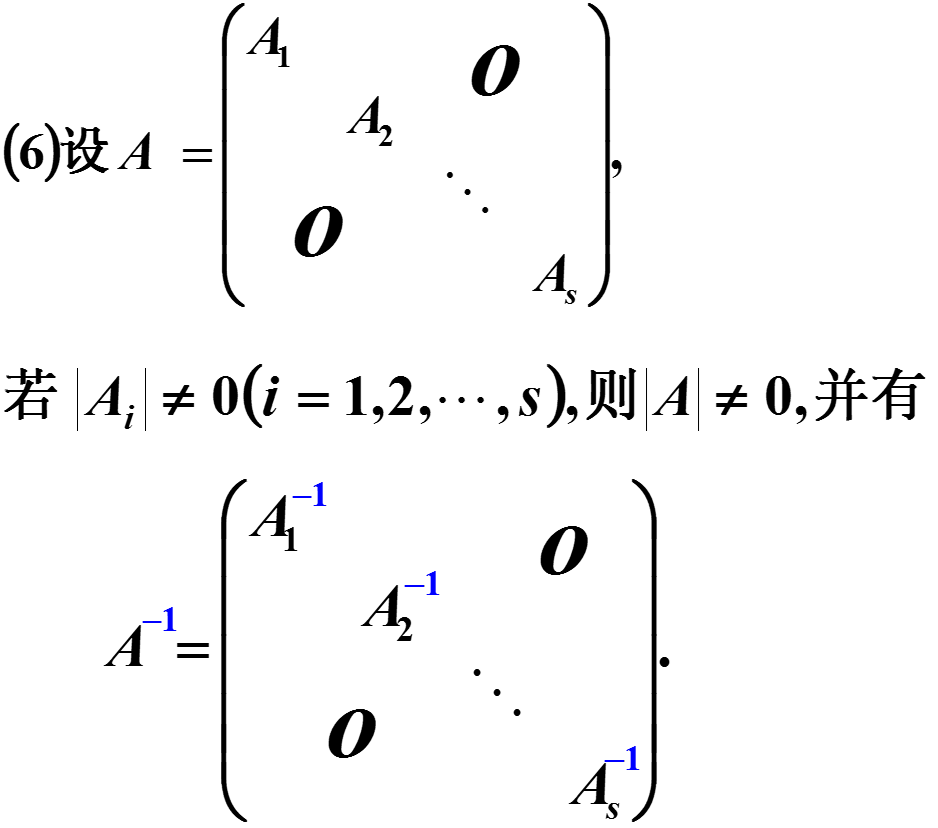## 向量• 私有
• 公开
• 删除